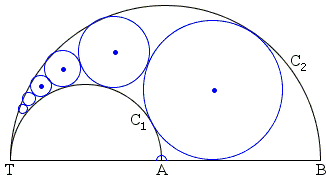# 7 = 2 + 5 Sangaku

A slew of sangaku problems deal with chains of inscribed circles, see, for example, Steiner's sangaku. The elegant sangaku below is the simplest in the collection by Fukagawa and Pedoe (1.8.6), yet it gives a chance to discuss a formula that was not used so far anywhere else at the site.The points T, A, B are collinear and TA = 2r and TB = 2s. The circles C1(r) and C2(s) have diameters TA and TB respectively. The circle O1(r1) touches AB, touches C1(r) externally and C2(s) internally, and we then form a chain of contact circles Oi(ri) (i = 1, 2, ...) as shown, all touching C1(r) externally and C2(s) internally. Show that

7 / r4 = 2 / r7 + 5 / r1.

Solution

### References

1. H. Fukagawa, D. Pedoe, Japanese Temple Geometry Problems, The Charles Babbage Research Center, Winnipeg, 1989

Write to:

Charles Babbage Research Center
P.O. Box 272, St. Norbert Postal Station
Winnipeg, MBThis sangaku dates from 1814 and was written in the Gumma prefecture.The points T, A, B are collinear and TA = 2r and TB = 2s. The circles C1(r) and C2(s) have diameters TA and TB respectively. The circle O1(r1) touches AB, touches C1(r) externally and C2(s) internally, and we then form a chain of contact circles Oi(ri) (i = 1, 2, ...) as shown, all touching C1(r) externally and C2(s) internally. Show that

7 / r4 = 2 / r7 + 5 / r1.

### Solution

The derivation of a more traditional formula for the common chain of circles inscribed in an arbelos

rn = ts / (n² + t + t²).

is easily adapted to the present case. The result is the following formula

 (*) rn = 4ts / ((2n - 1)² + 4t + 4t²),

where t = r / (s - r). Denoting S = 4t + 4t² we can write

• r1 = 4s / (1 + S),
• r4 = 4s / (7² + S),
• r7 = 4s / (13² + S),

which we plug into the required identity

7 / r4 = 2 / r7 + 5 / r1

getting

7(7² + S) = 2(13² + S) + 5(1 + S)

which simplifies to

7·7² = 2·13² + 5.

As one can easily verify, both sides are equal to 343.## Sangaku